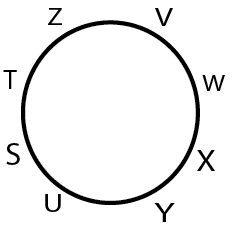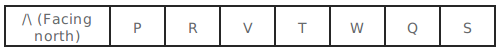0
10688

# Linear & Circular Arrangement Questions for SBI Clerk PDF:

Download Linear & Circular Seating Arrangement Questions and Answers for Banking exams of SBI Clerk & PO exam PDF.

Instructions (1 – 5): Study the information carefully and answer the questions:

S, T, U, V, W, X, Y and Z are sitting around the circle area, with equal distance amongst each other but not necessarily in the same order. Only two people face the centre and rest face outside (i.e.,in a direction opposite to the centre). Y sits second to left of W. S sits second left of Y. Only one person sits between S and z. T sits immediate next to S. T is not an immediate neighbour of Y. V is not an immediate neighbour of Y. Both the immediate neighbours of X face the centre.

Question 1: Who is sitting to immediate right of Z ?

a) Y
b) V
c) T
d) X
e) W

Question 2: Which of the following is true regarding U as per the given seating arrangement ?

a) X sits second to the left of U
b) Only three people sits between U and Y
c) Z is one of immediate neighbour of U
d) U faces the centre
e) S sits to immediate left of U

Question 3: What is T’s position with respect of Y ?

a) second to the right
b) second to the left
c) fifth to the left
d) fourth to the right
e) third to the left

Question 4: Which of the following group represents the immediate neighbours of X ?

a) WY
b) VX
c) TZ
d) VZ
e) SU

Question 5: Four of the following five are alike in a certain way based on the given seating
arrangement and so form a group. Which is the one does not belong to that group ?

a) Z
b) T
c) Y
d) V
e) X

Instructions (6 – 10): Study the following information answer the given questions:
P, Q, R, S, T, V and W are sitting in a straight line facing north but not necessarily in the same order. R sits third to the left of W. Neither R nor W sits at extreme ends of the line. Only one person sits between R and T. S is not an immediate neighbour of either R or W.Two people sit between T and P. Q is an immediate neighbour of S.

Question 6: Who amongst the following sits exactly between R and T ?

a) P
b) Q
c) V
d) S
e)None of these

Question 7: How many persons are sitting between P and W ?

a) One
b) Two
c) Three
d) Four
e) More than four

Question 8: What is the position of T with respect to Q ?

a) Third to the left
b) Fourth to the right
c) Second to the right
d) Second to the left
e) Immediate left

Question 9: Which of the following pairs represents persons sitting at two extreme ends of the line ?

a) S, V
b) P, S
c) T, V
d) P, Q
e) P, T

Question 10: Which of the following is true with respect to the given arrangement ?

a) Two people sit to the left of R
b) S sits to the immediate left of Q
c) W and V sit between T and P
d) Only one person sits to the right of S
e) None is true

Answers and Solutions for Linear Arrangement Questions for SBI Clerk PDF:

Solutions for (1 – 5):8 people S, T, U, V, W, X, Y and Z are sitting across a circular table and according to the given information order of their seating is given in the below diagram where W and Y are facing to the center and remaining are facing outwards to the center. Hence, V is the immediate neighbour of Z.

8 people S, T, U, V, W, X, Y and Z are sitting across a circular table and according to the given information order of their seating is given in the below diagram where W and Y are facing to the center and the remaining are facing outwards to the center. Hence, X is sitting 2nd to the left of U

8 people S, T, U, V, W, X, Y and Z are sitting across a circular table and according to the given information order of their seating is given in the below diagram where W and Y are facing to the center and the remaining are facing outwards to the center. Hence, Y is sitting 3rd left to T (As they are facing outward from the centre)

8 people S, T, U, V, W, X, Y and Z are sitting across a circular table and according to the given information order of their seating is given in the below diagram where W and Y are facing to the center and the remaining are facing outwards to the center. Hence, neighbours of X are W and Y

Z, T, V and X are facing towards outwards from the center but Y is facing towards the center hence, answer will be C.

Solutions (6 – 10):
The data given to us is:
P, Q, R, S, T, V and W are sitting in a straight line facing north but not necessarily in the same order. R sits third to the left of W. Neither R nor W sits at extreme ends of the line. Only one person sits between R and T. S is not an immediate neighbour of either R or W.Two people sit between T and P. Q is an immediate neighbour of S.

This data can be represented in diagrammatic form as: